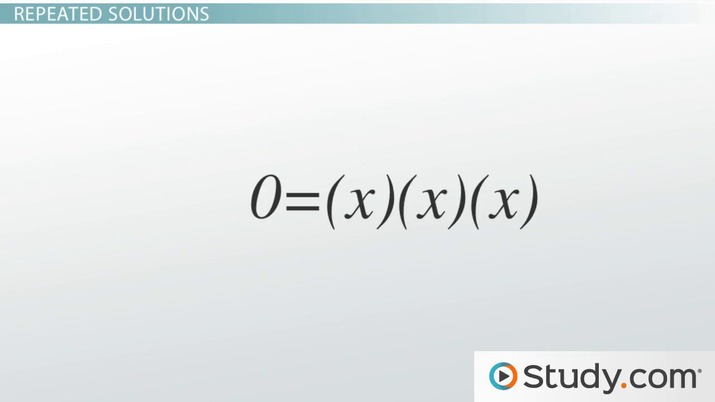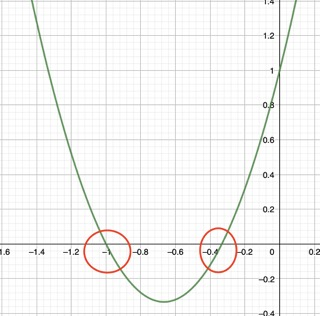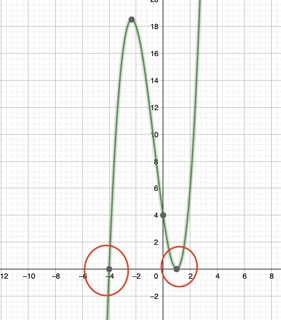# Fundamental Theorem of Algebra

Megan Krance, David Liano
• Author
Megan Krance

Megan has tutored in middle school level mathematics and high school level Algebra, Geometry, and Calculus for six years. They have a Bachelor's of Science Degree in Applied Mathematics from Robert Morris University in Moon Township, PA.

• Instructor
David Liano

David has a Master of Business Administration, a BS in Marketing, and a BA in History.

Understand what the definition of fundamental theorem of algebra is. See the proof for the fundamental theorem of algebra with examples`of algebra theorems. Updated: 01/11/2022

Show

## What is the Fundamental Theorem of Algebra

The definition of the fundamental theorem of algebra is: any polynomial of degree n has n roots. It also states that any polynomial has at least one solution. The theorem does not reveal what the roots of a polynomial are, but it does reveal how many there are. It takes basic mathematical skills to solve what the roots are; the theorem only states how many roots are in a polynomial. It also assists in solving polynomial equations, such as x^2-9=0. In the equation, x^2-9=0, use the definition of the fundamental theorem of algebra to find the number of roots.

x^2-9=0

add 9 to both sides

x^2=9

take the square root of both sides

x=\sqrt{9}

since 9 has a square root the solution is

x= +3,-3

The fundamental theorem of algebra says that any polynomial with n degree has n roots. This polynomial had 2 degrees and it was solved to show it has 2 roots.An error occurred trying to load this video.

Try refreshing the page, or contact customer support.

Coming up next: Using Rational & Complex Zeros to Write Polynomial Equations

### You're on a roll. Keep up the good work!

Replay
Your next lesson will play in 10 seconds
• 0:01 Bank Fee Analogy
• 0:48 Fundamental Theorem of Algebra
• 2:06 Imaginary Solutions
• 3:01 Repeated Solutions
• 3:55 Examples
• 6:58 Lesson Summary
Save Save

Want to watch this again later?

Timeline
Autoplay
Autoplay
Speed Speed

## The Roots of a Polynomial

Polynomial is derived from the Greek words "poly" meaning many and "nominal" meaning terms. A polynomial is a mathematical expression that contains two or more algebraic expressions such as constants, variables, and exponents that are combined by addition, subtraction, multiplication, and division. Polynomial degrees are defined as being the highest power of a variable in the equation or expression. For example, if a polynomial expression is 7x^5 + 3x^2 +4, the polynomial degree would be 5, since it is the highest degree throughout the entire polynomial. Polynomial roots or polynomial zeros are numbers that result in a polynomial function equaling zero. For example, a polynomial function is P(x)= 3x^2 +4x+1

P(-1/3)= 3(-1/3)^2+4(-1/3)+1

= 3(1/9)-4/3+1

=3/9-4/3+1

=3/9-12/9+9/9

=0

P(-1)=3(-1)^2+4(-1)+1

=3-4+1

=0

Graph the polynomial: P(x)=3x^2+4x+1The red circles on the graph point out the two roots of the polynomial. By the fundamental theorem of algebra, it is understood that by looking at the function, it will have 2 roots. Using basic math skills and setting the function equal to zero, we were able to find its polynomial roots or polynomial zeros. On the graph, its zeros are where the polynomial intersects with the x axis.

### Real and Complex Roots

A real number is any number that can be found on the number line going towards infinity. Examples of real numbers are whole numbers such as 0, -2, or 4, decimals such as 6.3, fractions such as -2/5, or even square roots such as \sqrt{14}. It should be noted that infinity is not a real number and neither is i, which is \sqrt{-1}. In the case of i, the reason it is not considered a real number is because it is an imaginary number. The \sqrt{-1} does not exist because there is no number whose square is equal to -1. Because of that, i is not regarded as a real number, but as a complex number instead. Complex numbers are any numbers that include the imaginary number i. Some examples of complex numbers are 5i, 2+7i, and 9.4i. Actually, real numbers can be considered a subset of complex numbers because a real number could be written as r + si, where r represents the real number and s represents the coefficient to the imaginary number. So, the real number 5 could be written as a complex number: 5 + 0i.

Because the fundamental theorem of algebra states that any polynomial of a degree n has n number of roots, it also means that a polynomial function has at least one root based on the complex number system. Roots of polynomials can be real or complex. For example, if the polynomial function is something like x^2+9, its 2 roots would be +3 and -3, which are real numbers. However, if the polynomial function is something like x^5 - x^4 + x^3 - x^2 - 12x + 12:

x^5 - x^4 + x^3 - 12x + 12 = 0

(x - 1)(x^4 + x^2 - 12) = 0 Factor out the equation

(x - 1)(x^2 - 3)(x^2 + 4) = 0

(x - 1)(x + \sqrt{3})(x - \sqrt{3})(x^2 + 4) = 0

(x - 1)(x + \sqrt{3})(x - \sqrt{3})(x + 2i)(x - 2i) = 0

This polynomial has 5 roots and 3 of them are real and 2 are complex.

A complex number comes in pairs due to the Complex Conjugate Root Theorem. It states that if one complex root is discovered, then its complex conjugate is also a root. This theorem means that if one complex root of a polynomial is a+bi then another root will be a-bi. If a complex root is given to a 3 root polynomial, then the second root is automatically known due to the complex conjugate root theorem.

Here is a table of degrees of Polynomials and their roots:

 Degrees of Polynomials Number of Roots 1 1 real 2 2 real or 2 complex 3 3 real or 1 real and 2 complex 4 4 real or 2 real and 2 complex or 4 complex

### Repeated Roots

When a function of a polynomial has the same solution twice, it has a repeated root. On a graph, a root is repeated when it touches or is tangent to the x axis; it does not pass through the x axis. For example, take the polynomial function:

P(x) = (x -1)^2 (x + 4)

(x -1)^2 (x + 4) = 0

(x + 4) = 0

X = -4

(x - 1)^2 = 0

Because it is squared, the solution to (x - 1)^2 = 0, which is x = 1, occurs twice and is considered a double root.On the graph, the one root, x = -4, passes through the x axis because it is a single, real root. However, the other solution of x = 1, which is a repeated root, does not pass through the x axis, it just touches it. When a polynomial is graphed, the roots that pass through the x axis are single, individual roots, the roots that are tangent to the x axis are repeated roots.

### Fundamental Theorem of Algebra Example 1

Let the function be P(x) = x^3 + 3x^2 - 4x

Using the fundamental theorem of algebra definition, any polynomial of degree n has n roots. x^3 is the variable with the highest power, so based on the fundamental theorem of algebra, this equation has 3 roots.

Simplify the equation by factoring:

x^3 + 3x^2 - 4x = 0

x(x^2 + 3x -4) = 0

x = 0

(x^2 + 3x - 4) = 0

(x + 4)(x - 1) = 0

To unlock this lesson you must be a Study.com Member.

#### How do you prove the fundamental theorem of algebra?

You prove the fundamental theorem of algebra by using the linear factorization theorem. By using that theorem, then allow a polynomial function, P, be written as P(x) = ax^n+ax^n-1+...a^0. Because of the definition of the linear factorization theorem, the polynomial can be factored as P(x) = (x - g_1)(x - g_2)....(x - g_n) where g_1, g_2, … g_n are complex numbers. Thus, any polynomial has exactly n solutions among the complex numbers.

#### What is the fundamental theorem of algebra used for in real life?

The fundamental theorem of algebra is used in real life as a structure for other algebraic and trigonometric areas of study. It is used also in linear algebra and algebraic geometry.

#### What is the fundamental theorem of algebra?

The fundamental theorem of algebra states that any polynomial of degree n has n roots. It also states that any polynomial has at least one solution. The theorem does not reveal what the roots of a polynomial are, but it does reveal how many there are.

### Register to view this lesson

Are you a student or a teacher?

Back

### Resources created by teachers for teachers

Over 30,000 video lessons & teaching resources‐all in one place.Video lessonsQuizzes & WorksheetsClassroom IntegrationLesson Plans

I would definitely recommend Study.com to my colleagues. It’s like a teacher waved a magic wand and did the work for me. I feel like it’s a lifeline.

Jennifer B.
TeacherCreate an account to start this course today
Used by over 30 million students worldwide